# Per Capita GDP by EU and US State, Second Quarter 2021

Nov 16, 2021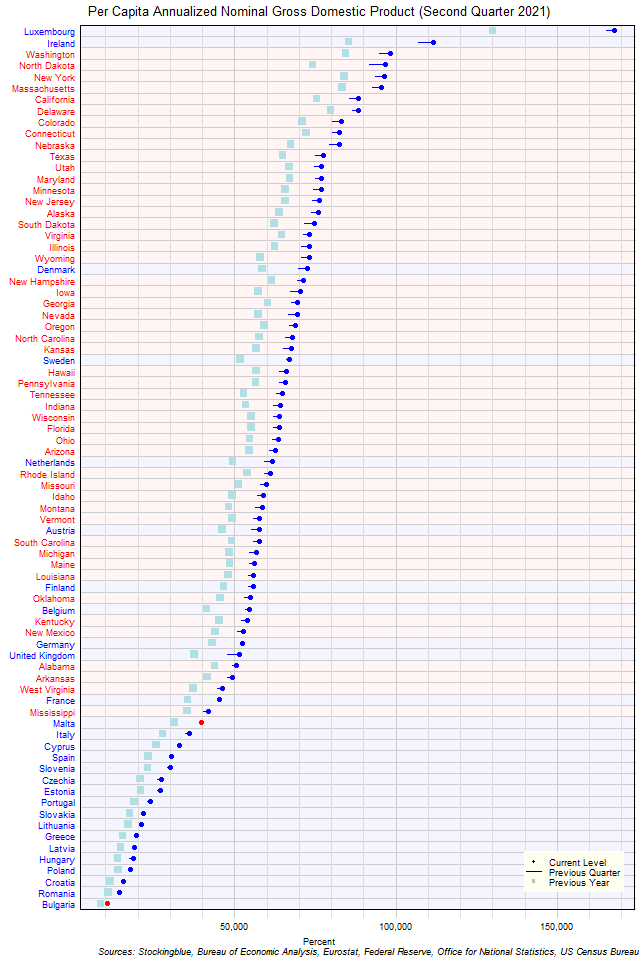The chart above shows the per capita annualized nominal gross domestic product (GDP) in each EU and US state as of the second quarter of 2021 in dollars, the change from the previous quarter, and the GDP one year prior.  Every single US state's per capita GDP grew over the past quarter.

# Per Capita GDP by EU State, Second Quarter 2021

Nov 15, 2021The chart above shows the per capita annualized nominal gross domestic product (GDP) in each EU state as of the second quarter of 2021 in euros, the change from the previous quarter, and the GDP one year prior.  Two states saw a contraction over the previous quarter.

# Per Capita GDP by US State, Second Quarter 2021

Nov 12, 2021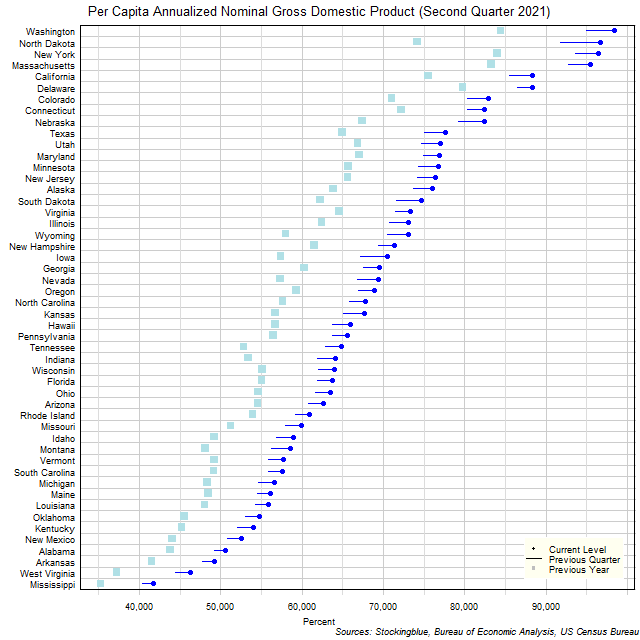The chart above shows the per capita annualized nominal gross domestic product (GDP) in each US state as of the second quarter of 2021 in dollars, the change from the previous quarter, and the GDP one year prior.  Every single state's economy grew over the past quarter.

# Long-Term Per Capita GDP by EU and US State, First Quarter 2021

Sep 16, 2021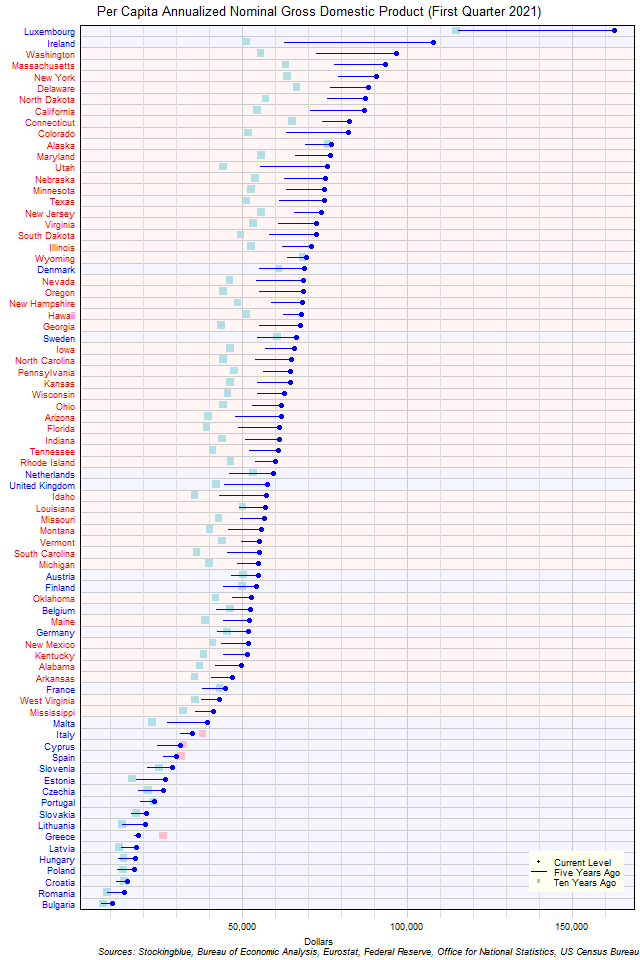The chart above shows the per capita annualized nominal gross domestic product (GDP) in each EU and US state as of the first quarter of 2021 in dollars, the change from five years ago, and the GDP ten years prior.  There are four states that have a lower per capita GDP now than ten years ago.

# Long-Term Per Capita GDP by EU State, First Quarter 2021

Sep 15, 2021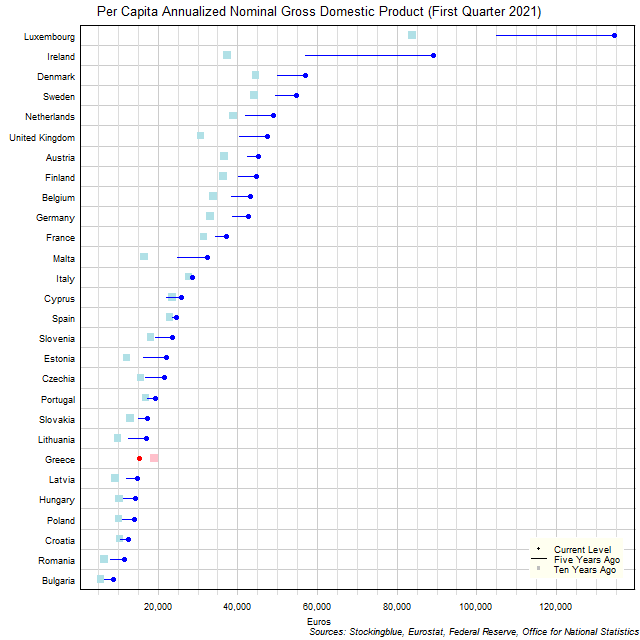The chart above shows the per capita annualized nominal gross domestic product (GDP) in each EU state as of the first quarter of 2021 in euros, the change from five years ago, and the per capita GDP ten years prior.  Luxembourg and Ireland had exceptional growth over the past five years.

# Per Capita GDP by EU and US State, First Quarter 2021

Jul 30, 2021The chart above shows the per capita annualized nominal gross domestic product (GDP) in each EU and US state as of the first quarter of 2021 in dollars, the change from the previous quarter, and the GDP one year prior.  Every single US state's per capita GDP grew over the past quarter.

# Per Capita GDP by EU State, First Quarter 2021

Jul 29, 2021The chart above shows the per capita annualized nominal gross domestic product (GDP) in each EU state as of the first quarter of 2021 in euros, the change from the previous quarter, and the GDP one year prior.  Six states saw a contraction over the previous quarter.

# Per Capita GDP by US State, First Quarter 2021

Jul 28, 2021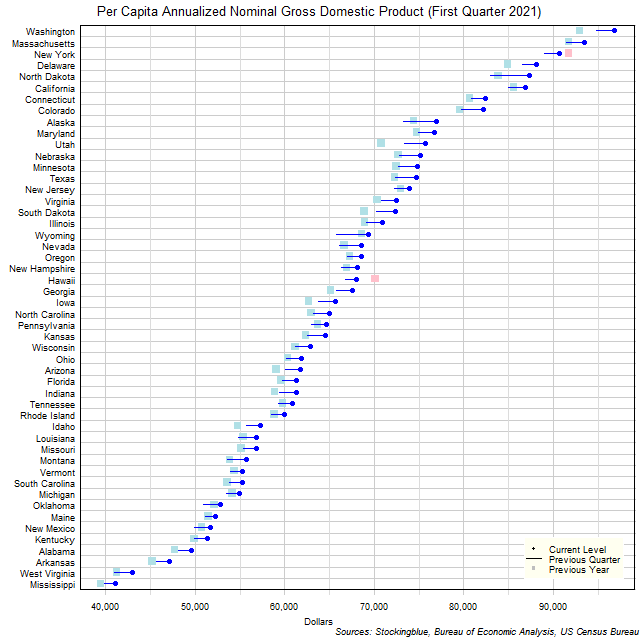The chart above shows the per capita annualized nominal gross domestic product (GDP) in each US state as of the first quarter of 2021 in dollars, the change from the previous quarter, and the GDP one year prior.  Every single state's economy grew over the past quarter.

# Long-Term Per Capita GDP by EU and US State, Fourth Quarter 2020

May 27, 2021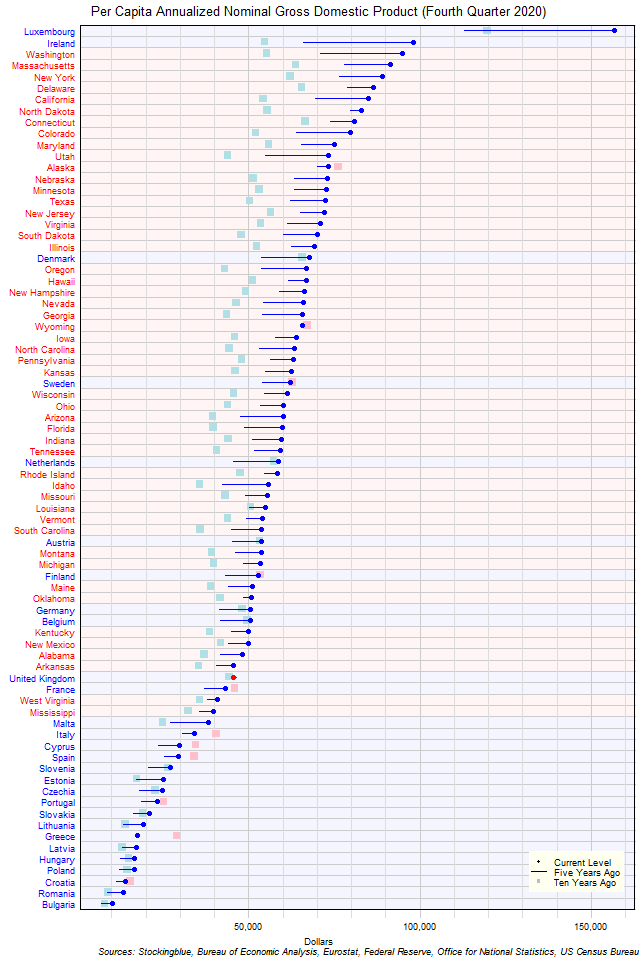The chart above shows the per capita annualized nominal gross domestic product (GDP) in each EU and US state as of the fourth quarter of 2020 in dollars, the change from five years ago, and the GDP ten years prior.  There is one state that has a lower per capita GDP now than five years ago and 11 that have a lower per capita GDP now than ten years ago.

# Long-Term Per Capita GDP by EU State, Fourth Quarter 2020

May 26, 2021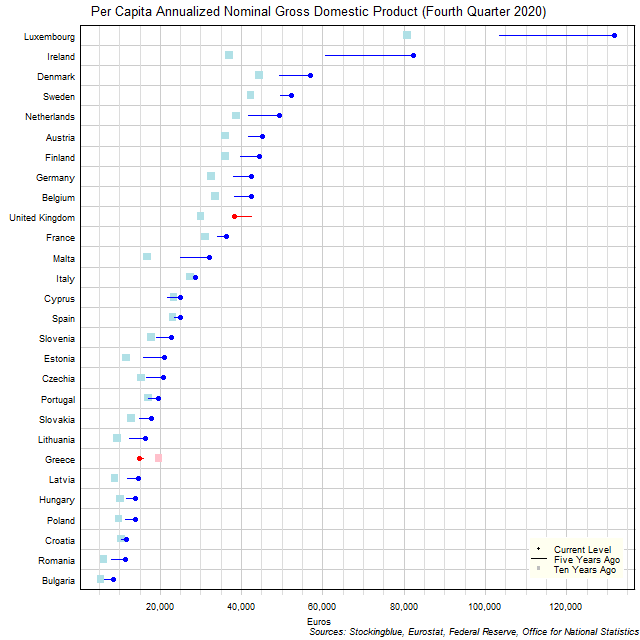The chart above shows the per capita annualized nominal gross domestic product (GDP) in each EU state as of the fourth quarter of 2020 in euros, the change from five years ago, and the per capita GDP ten years prior.  Luxembourg and Ireland had exceptional growth over the past five years.

Older